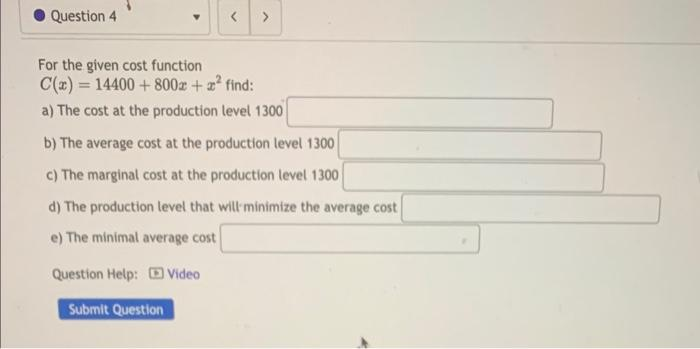Home / Expert Answers / Calculus / for-the-given-cost-function-c-x-14400-800x-x2-find-a-the-cost-at-the-production-level-1300-b-th-pa252

# (Solved): For the given cost function C(x)=14400+800x+x2 find: a) The cost at the production level 1300 b) Th ...For the given cost function find: a) The cost at the production level 1300 b) The average cost at the production level 1300 c) The marginal cost at the production level 1300 d) The production level that wiltminimize the average cost e) The minimal average cost Question Help: [ Video

We have an Answer from Expert